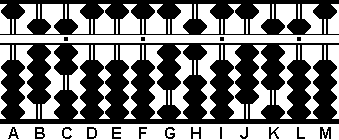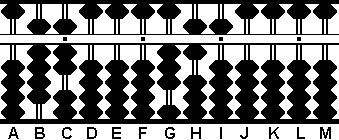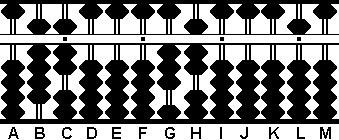###Division Revision

When solving problems of division on a soroban, sometimes arriving at the correct answer can involve a little trial and error. The following is some correspondence I had with one of our group member's who was having difficulty tackling a division problem. The problem is a great example of how quickly and easily one can revise quotient answers.

##### SR

July 21, 2004

Hi SR - Let's see if I can help you to see the procedure for doing this problem.

Your problem: 35405 ÷ 97 = 365

Step 1: Set the dividend 35405 on HIJKL and  the divisor 97 on BC (Fig.1)Fig. 1 `Step 1` ```A B C D E F G H I J K L M . . . .   0 9 7 0 0 0 0 3 5 4 0 5 0 ```

Step 2: The first number in the quotient will be 3. Place 3 on rod G.

2a: Multiply 3 on G by 9 on B and subtract the product 27 from 35 on rods HI.

2b: Multiply 3 on G by 7 on C and subtract the product 21 from rods IJ. This leaves the partial quotient 3 on rod G and the remainder 6305 on rods IJKL. (Fig.2)Fig. 2 `Step 2` ```A B C D E F G H I J K L M . . . .   0 9 7 0 0 0 0 3 5 4 0 5 0 (3) Step 2 - 2 7 Step 2a 0 9 7 0 0 0 0 0 8 4 0 5 0 - 2 1 Step 2b 0 9 7 0 0 0 3 0 6 3 0 5 0```

Step 3: The next number in the quotient will be 6. Place 6 on rod H.

3a: Multiply 6 on H by 9 on B and subtract the product 54 from 63 on rods IJ.

3b: Multiply 6 on H by 7 on C and subtract the product 42 from rods JK, leaving the partial quotient 36 on rods GH and the remainder 485 on rods JKL. (Fig.3)Fig. 3 `Step 3` ```A B C D E F G H I J K L M . . . .   0 9 7 0 0 0 3 0 6 3 0 5 0 (6) Step 3 - 5 4 Step 3a 0 9 7 0 0 0 3 0 0 9 0 5 0 - 4 2 Step 3b 0 9 7 0 0 0 3 6 0 4 8 5 0```

Step 4: The next number in the quotient will be 5. Place 5 on rod I.

4a: Multiply 5 on I by 9 on B and subtract the product 45 from rods JK

4b: Multiply 5 on I by 7 on C and subtract the product 35 from rods KL. This leaves the answer 365 on rods GHI (Fig.4)Fig. 4 `Step 4` ```A B C D E F G H I J K L M . . . .   0 9 7 0 0 0 3 6 0 4 8 5 0 (5) Step 4 - 4 5 Step 4a 0 9 7 0 0 0 3 6 0 0 3 5 0 - 3 5 Step 4b 0 9 7 0 0 0 3 6 5 0 0 0 0 ```

Hope this helps,

Totton Heffelfinger

##### At 09:24 PM 7/21/2004 -0700, SR wrote: Hello sir,   Thank you very much for your help in doing the problem, but I still have a doubt. In the problem in Step 3 the quotient is taken as 6. But we can take it as 7 as 63=9*7. I was stuck at this stage. So, I'll be glad if I can get a reason why 6 is taken as the Quotient.   Thanking you.   Regards, SR.

July 22, 2004

Hi SR - This is a problem many people struggle with when they first begin doing long division on both paper *and* the abacus. And this is where the abacus really is a fun tool for doing division problems because it allows us to quickly *revise* a wrong answer. You'll see what I mean.

(Let's start again from the beginning but this time we'll see if we can clear up your doubts.)

Your problem: 35405 ÷ 97 = 365

Step 1: Set the dividend 35405 on HIJKL and  the divisor 97 on BC (Fig.1)Fig. 1 `Step 2` ```A B C D E F G H I J K L M . . . .   0 9 7 0 0 0 0 3 5 4 0 5 0 ```

Step 2:
The first number in the quotient will be 3. Place 3 on rod G.

2a: Multiply 3 on G by 9 on B and subtract the product 27 from 35 on rods HI.

2b: Multiply 3 on G by 7 on C and subtract the product 21 from rods IJ. This leaves the partial quotient 3 on rod G and the remainder 6305 on rods IJKL. (Fig.2)Fig. 2 `Step 2` ```A B C D E F G H I J K L M . . . .   0 9 7 0 0 0 0 3 5 4 0 5 0 (3) Step 2 - 2 7 Step 2a 0 9 7 0 0 0 0 0 8 4 0 5 0 - 2 1 Step 2b 0 9 7 0 0 0 3 0 6 3 0 5 0```

Okay. This is where you're having difficulty. From here, let's say we follow your suggestion and do Step 3 using a quotient of 7. We would have the following;

Step 3: The next number in the quotient will be 7. Place 7 on rod H.

3a: Multiply 7 on H by 9 on B and subtract the product 63 from 63 on rods IJ.

3b: Now, following the rules for division, we have to multiply 7 on H by 7 on C and subtract the product 49 from rods JK  ....but here's the problem. We don't have 49 available on rods JK. In fact all we have is 00. And following the rules of division we must have enough to multiply and subtract again. In other words the quotient 7 was too large. In essence the mistake was this; we've taken away 09 one too many times  from rods IJ. (Fig.3)Fig. 3 `Step 3` ```A B C D E F G H I J K L M . . . .   0 9 7 0 0 0 3 0 6 3 0 5 0 (7) Step 3 - 6 3 Step 3a 0 9 7 0 0 0 3 7 0 0 0 5 0 - 4 9 Step 3b can't 0 9 7 0 0 0 3 7 0 0 0 5 0 be done! ```

THE SOLUTION:

Here's where we can *revise* our answer quickly and easily.

Step 4:  Subtract 1 from the the quotient 7 on rod H leaving 36 on rods GH.

4a: Because we subtracted 1 from the quotient we have to adjust the dividend as well. Do this by *adding* 09 to rods IJ. This leaves the partial quotient 36 on rods GH and the remainder 905 on rods JKL. (Fig.4)Fig. 4 `Step 4` ```A B C D E F G H I J K L M . . . .   0 9 7 0 0 0 3 7 0 0 0 5 0 -(1) Step 4 0 9 7 0 0 0 3 6 0 0 0 5 0 + 0 9 Step 4a 0 9 7 0 0 0 3 6 0 9 0 5 0 ```

Now that we've *revised* our answer we can continue. *But* this time instead of multiplying by the quotient 7 on H (our mistake) we continue by multiplying the *revised* quotient 6.

Step 5: Multiply 6 on H by 7 on C and subtract the product 42 from rods JK.  As you can see this time we have enough on rods JK because 42 can be nicely subtracted from 90. This  leaves the partial quotient 36 on rods GH and the remainder 485 on rods JKL. (Fig.5)Fig. 5 `Step 5` ```A B C D E F G H I J K L M . . . .   0 9 7 0 0 0 3 6 0 9 0 5 0 (6) - 4 2 Step 5 0 9 7 0 0 0 3 6 0 4 8 5 0 ```

Step 6:
The next number in the quotient will be 5. Place 5 on rod I.

6a: Multiply 5 on I by 9 on B and subtract the product 45 from 48 on rods JK

6b and the answer: Multiply 5 on I by 7 on C and subtract the product 35 from rods KL. This leaves the answer 365 on rods GHI (Fig.6)Fig. 6 `Step 6` ```A B C D E F G H I J K L M . . . .   0 9 7 0 0 0 3 6 0 4 8 5 0 (5) Step 6 - 4 5 Step 6a 0 9 7 0 0 0 3 6 0 0 3 5 0 - 3 5 Step 6b 0 9 7 0 0 0 3 6 5 0 0 0 0 ```

If still having trouble why not simplify the question somewhat? Try it this way;

6305 ÷ 97 =

Other examples you might try are;

6324 ÷ 93 =
5481 ÷ 63 =
4964 ÷ 73 =

- Totton Heffelfinger